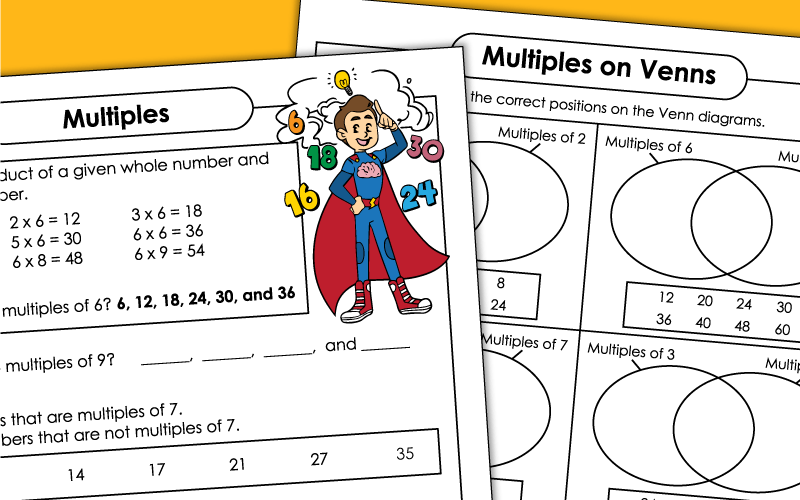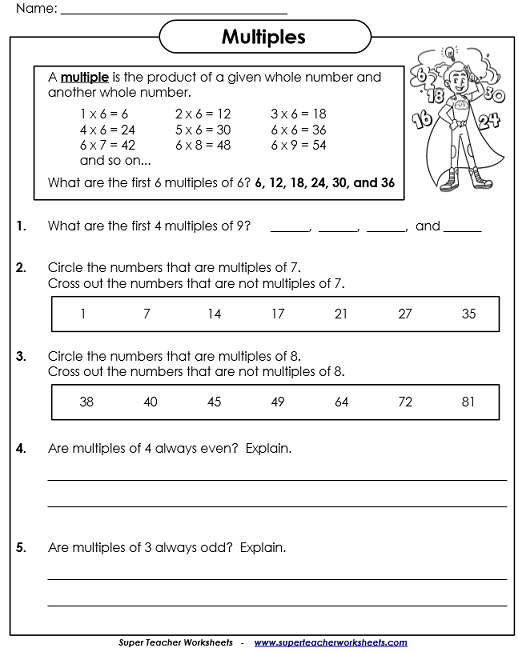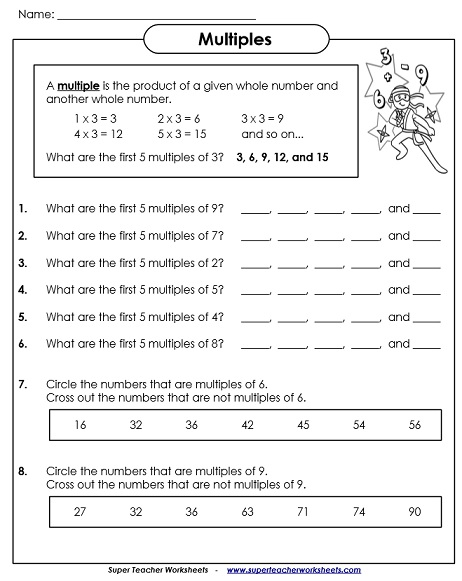# Multiples## Worksheets

On this printable, students will list multiples of numbers. Then there are two short answer questions.
Part 1: List the first five multiples of the given numbers. Part 2: Circle the multiples of the given number.
Place the numbers in the correct place on the Venn diagrams. (For example: There's a Venn diagram with "Multiples of 3" and "Multiples of 2." Students place the given numbers in the correct place on the Venn.)
This is similar to the other Venn diagram worksheet except it includes more complex versions of Venn diagrams.
On this diagram, students sort numbers by into one of these groups: multiples of 3, multiples of 5, and multiples of 7. They also sort by odd and even.
Least Common Multiple Worksheets

Here you'll find worksheets on calculating the least common multiple, or LCM.

Factoring and GCF Worksheets

Find factors of numbers, as well as the greatest common factor.

## ImagesMy Account
Site Information